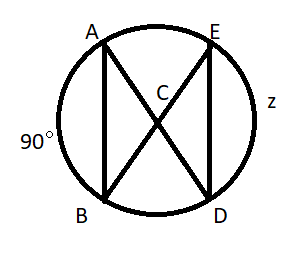Filters
Latest Questions
Mathematics
Congruent arcs
State true or false.
If the angles subtended by the chords of a circle at the center are equal , then the chords are equal.

Mathematics
Congruent arcs
In the circle shown below, AB = 3 and DE = 3. What is the value of z?Prev
1
Next